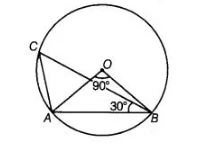# In figure, if ∠AOB = 90°`
Question:

In figure, if ∠AOB = 90° and ∠ABC = 30°, then ∠CAO is equal to(a) 30°

(b) 45°

(c) 90°

(d) 60°

Solution:

(d)

In AOAB, ∠OAB + ∠ABO + ∠BOA = 180°

∠OAB + ∠OAB + 90° = 180° => 2∠OAB = 180°- 90°

[angles opposite to equal sides are equal] [angle sum property of a triangle] [from Eq. (i)]

=> ∠OAB = 90°/2 = 45° …(i)

In ΔACB, ∠ACB + ∠CBA + ∠CAB = 180°

∴ 45°+ 30°+ ∠CAB = 180°

=> ∠CAB = 180° – 75° = 105°

∠CAO+ ∠OAB = 105°

∠CAO + 45° = 105°

∠CAO = 105° – 45° = 60°